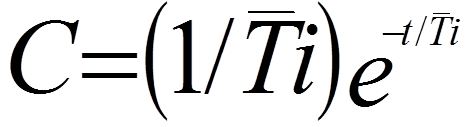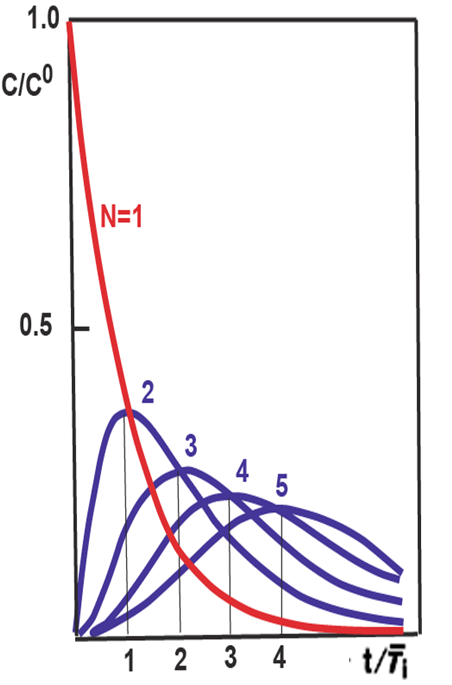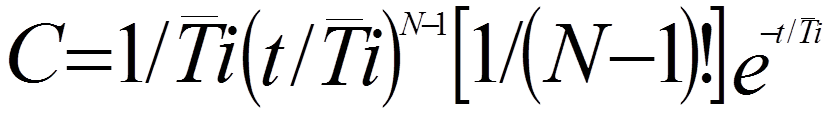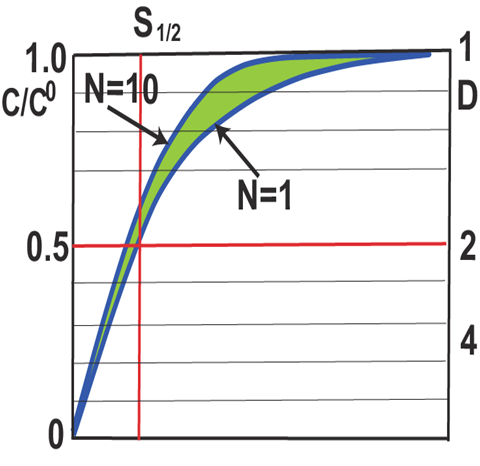0.3.4.
Tank in Series ModelWhen  an initially square input passes through a series of ideally stirred tanks it will form a gradient, the shape of which changes from exponential (N=1) to Gaussian(N=10). The parameters of this model are N- the number of mixing stages, through which the element of fluid has passed, and  the mean residence time of the element of in one mixing stage. For one tank the normalized C curve is:A
while for system with any number of serial mixing stages:B
applies the statistical G function, that for large N approaches Gaussian shape (A). The F curve (B) for the same serial passage changes from exponential (N=1) to erf function (N=10). The green shaded area covers the range of FI responses obtained with increased intensity of radial mass transfer.